# Integral of e^-x^t using Feynman’s technique

Wouldn’t it be nice to generalize the Gaussian integral to any exponent of –x? This is the famous integral: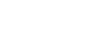Since this is an even function, it can be written as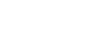Therefore, half of this integral will be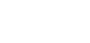What we want to do today is parameterize it in order to find a formula for any exponent of the -x; we are going to use the parameter t: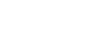But why aren’t we using this: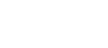?

The answer is simple: if the bounds of integration are symmetrical, i.e. -a and a, for odd functions the integral will give 0. The function that we have becomes odd for odd values of t and so we won’t be generalizing it to any number, since we’ll get 0 everytime t is an odd number.

So, this is the integral we are interested in:I came up with a very beautiful way to solve this: differentiation under the integral sign! In my last post I showed you how to evaluate the integral from 0 to infinity of sin(x)/x using this approach, so if you want, go and check it out to become more familiar with the concept!

If we could have this form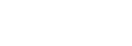it would be very easy to evaluate by performing a substitution: if we let u=x^t, the derivative will be tx^(t-1) and the x(t-1) will cancel out. We need to find a way to generate that term.

What if we introduce a new parameter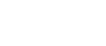so that, when taking the derivative, x^t will be generated? This would be very similar to the x^(t-1) we wanted; then, with a substitution, we will be able to simplify things. Let’s do this! But first, we need to write the original integral is some sort of way. We will write the general one as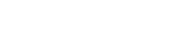You can see that the original one has a=1, therefore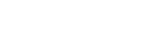This will be useful later on.

Let’s start differentiating!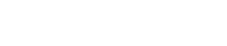We can move the derivative inside the integral, but it becomes partial derivative since we are differentiating only one term of the sum (which is given by the integral of the function; again, I explain this here):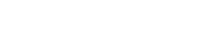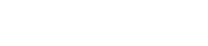At this point we can perform an s substitution (you can use whatever letter you want). You’ll be better off letting s=ax^t instead of s=-ax^t as this will need another substitution that may not be very intuitive. I actually used the second substitution, but then found that letting s=ax^t is faster. However, I will show you both ways.

Substitution with s=ax^t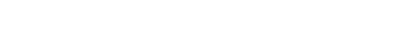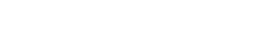Let’s bring the constant to the front of the integral (linearity):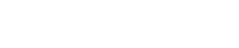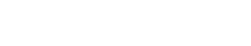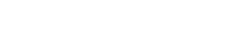Can you see where the problem is? We are integrating with respect to s, but we still have an x. If you remember, though, we let s=ax^t, so we can find the x and write it in terms of the current variable of integration s. I explain this way of proceeding here;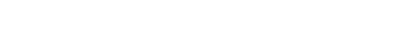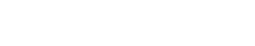Now we are in the s “world”. Again, let’s take out the constants:Can you see it? This looks a lot like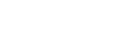It’s almost identical! n-1 is 1/t and, guess what? That’s the gamma function!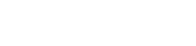and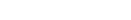Therefore,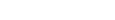and look at this: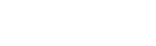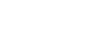1/t is our n! (the ‘!’ here does not mean “factorial” lol). This means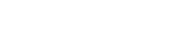or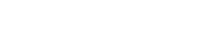Hence,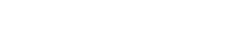Substitution using s=-ax^t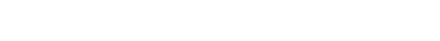Now the bounds of integration change: the lower bound is s1=-a*0=0, the upper one is s2=-a*infinity=-infinity. Before, nothing changed because s1 and s2 where still 0 and infinity.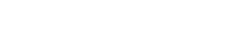Let’s bring the constant to the front of the integral (linearity):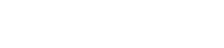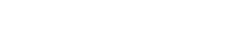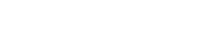Same as before: let’s find x: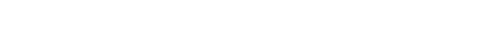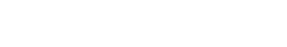Now we are in the s “world”. Again, let’s take out the constants: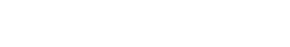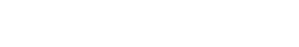This also looks like the gamma function, except that we have e^s instead of e^-s. We can remedy by letting u=-s, and so du=-ds (and so ds=-du, or ds=(-1)du); remember that the bounds change accordingly: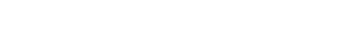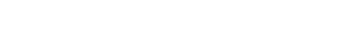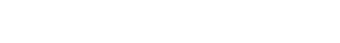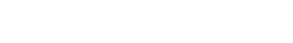(-1)^(2/t) is the same as ((-1)²)^(1/t), therefore it gives simply 1.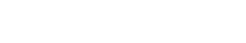As we saw before, this becomes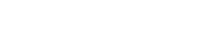And this is exactly what we got from the other substitution!

Now we can find I(a). How? Taking the integral on both sides, since I'(a) is the derivative: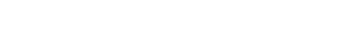Constants out: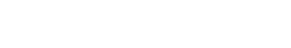Let’s apply the power rule: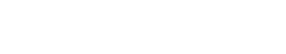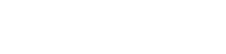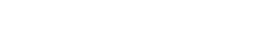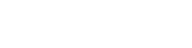Now we can add the constant C: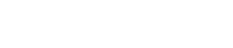Like we did in the previous post, we must find the value of C. To do so, let’s first rewrite the integral: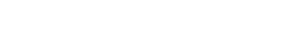How can we make the integral equal to 0? If we let a = infinity, the integral becomes 0! This is because e^infinity=0.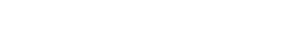Infinity raised to a negative number is equal to 0, because: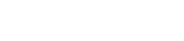therefore, thisbecomesand so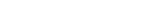Let’s rewrite the integral: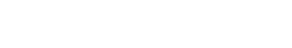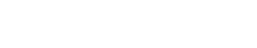Now, since we had a=1 at the beginning, we will plug in 1 to find the answer: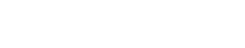1 raised to any number is still equal to 1, hence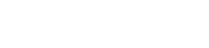And this is the answer! You can also write it like this: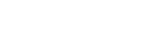Thank you so much for reading! If you liked this post give it a like, it would mean a lot to me! Also, subscribe if you want to receive notifications whenever I post something! If you have any doubts, questions, suggestions, leave a comment and I’ll be happy to reply!

Join 31 other subscribers.